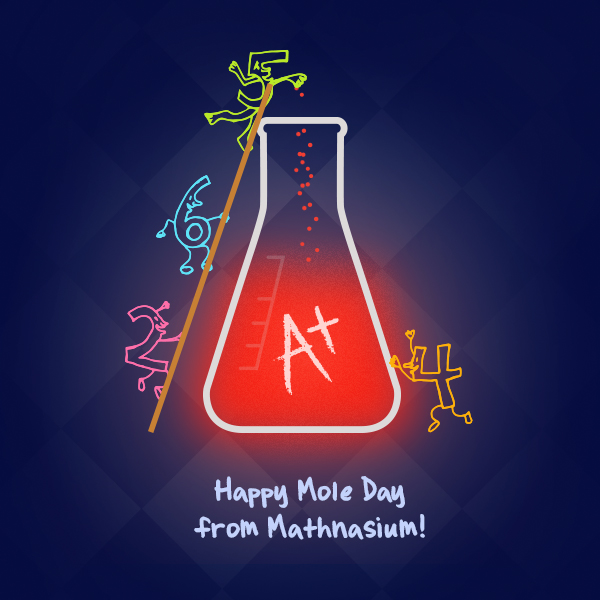Holy Moley! October 23 is National Mole Day!

By Damaris | Added Oct 23, 2015It’s a big day for chemistry (and math!) enthusiasts—October 23 is National Mole Day! Before images of burrowing, subterranean rodents with limited vision come to mind, allow us to offer a brief explanation of this very big number.

In chemistry, a mole is a unit of measurement that describes a quantity of anything! While you could conceivably have a mole of water molecules, a mole of chocolates, or a mole of whales—anything countable, really—a mole is a very, very large quantity. One mole is equal to 6.022 × 1023 particles of the substance you’re counting. That’s a lot of stuff, which is why the mole is used by chemists exclusively in reference to things that are very small, such as the number of molecules of chemical compounds or the number of atoms of a given element.

6.022 x 1023 is referred to as Avogadro’s Number, named for 19th century Italian chemist Amedeo Avogadro, whose theories laid the groundwork for atomic theory.

For a fun, quick lesson on the mole and its history, check out this awesome video (via TED-Ed):

Here’s a quick example of how chemists use the mole in calculations.

How many molecules are there in 36 grams of H2O?

Solution: To get from grams to molecules, first we have to go from grams to moles. Then we convert from moles to grams.

A mole is equal to the mass of 6.022 × 1023 particles of a given element. Hydrogen has a molar mass of 1 gram per mole (1 amu) and oxygen has a molar mass of 16 grams per mole. That said, H2O has a molar mass of (1 × 2) + (16 × 1) = 18 grams per mole.

So, 36 grams of H2O × (1 mole ÷ 18 grams) = 2 moles of H2O

To go from moles to molecules, we multiply by Avogadro's number, 6.022 × 1023.

So, 2 moles of H2O × 6.022 × 1023 = 1.204 × 1023 = 1.204 × 1024. (High school chemistry students—don’t forget your significant figures!)

Therefore, there are 1.204 × 1024 molecules of H2O in a 36-gram sample!

Now try this: How many grams of CH4 are in a sample containing 1.8066 × 1024 molecules of CH4? Email your answer to ilovemath@mathnasium.com for a chance to win a Mathnasium prize pack!